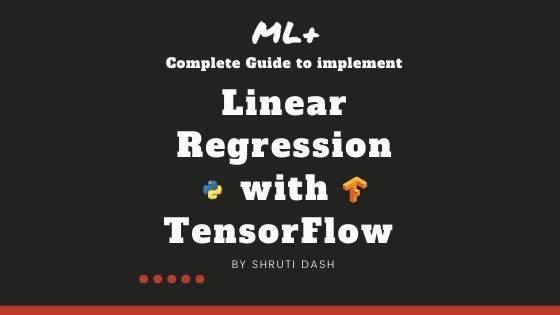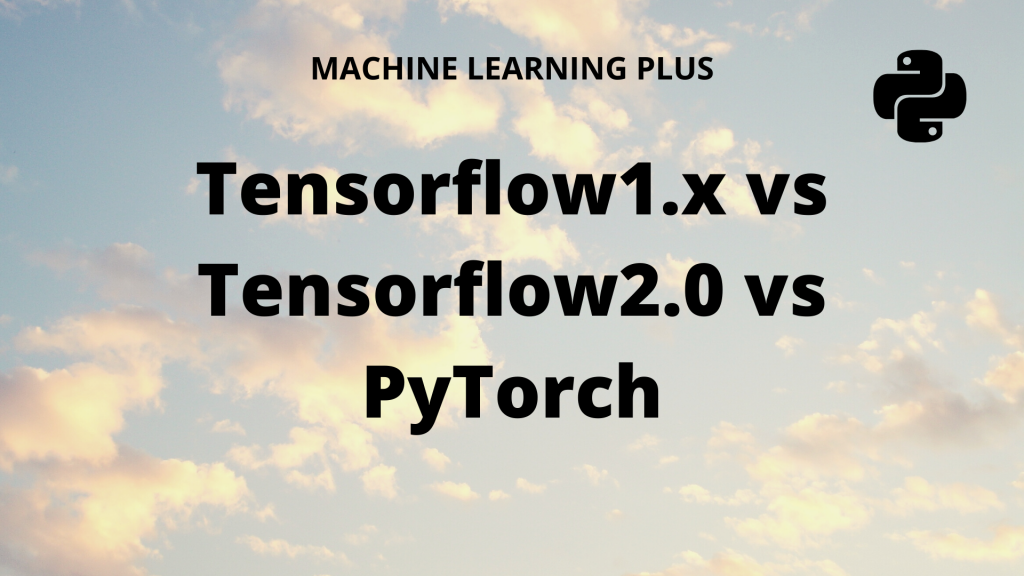Drop a Query

# Deep Learning## How to implement Linear Regression in TensorFlow

Linear Regression is one of the fundamental machine learning algorithms used to predict a continuous variable using one or more explanatory variables (features). In this tutorial, you will learn how to implement a simple linear regression in Tensorflow 2.0 using the Gradient Tape API. Overview In this tutorial, you will understand: Fundamentals of Linear Regression …

## How to use tf.function to speed up Python code in Tensorflow

tf.function is a decorator function provided by Tensorflow 2.0 that converts regular python code to a callable Tensorflow graph function, which is usually more performant and python independent. It is used to create portable Tensorflow models.       Introduction Tensorflow released the second version of the library in September 2019. This version, popularly called …## TensorFlow vs PyTorch – A Detailed Comparison

Compare the popular deep learning frameworks: Tensorflow vs Pytorch. We will go into the details behind how TensorFlow 1.x, TensorFlow 2.0 and PyTorch compare against eachother. And how does keras fit in here. Table of Contents: Introduction Tensorflow: 1.x vs 2 Difference between static and dynamic computation graph Keras integration or rather centralization What is …Course Preview

## Machine Learning A-Z™: Hands-On Python & R In Data Science

### Free Sample Videos:#### Machine Learning A-Z™: Hands-On Python & R In Data Science#### Machine Learning A-Z™: Hands-On Python & R In Data Science#### Machine Learning A-Z™: Hands-On Python & R In Data Science#### Machine Learning A-Z™: Hands-On Python & R In Data Science